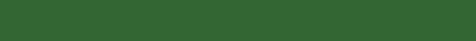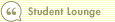DuPont and ROEIn this course1 Introduction 2 Free Cash Flow 3 Profit Margins 4 Turnover 5 Return on Equity and Assets 6 DuPont Equation 7 Margin vs. Turnover 8 DuPont and ROE 9 Return on Invested Capital 10 The Bottom LineTo use the DuPont equation to calculate a company's ROE, we have to add a step to the process to account for the amount of leverage (debt) a company employs. We can break down ROE using the DuPont equation as follows:

ROE = ROA x (Asset / Equity Ratio)

ROE = (Asset Turnover) x (Net Profit Margin) x (Asset / Equity Ratio)

ROE = (Sales / Average Assets) x (Net Profits / Sales) x (Average Assets / Average Equity)

=

(Net Profits) / (Average Equity)

Notice that in the ROE breakdown, both sales and average assets cancel each other out.

One can draw the same insights about operating efficiency (profit margins) and asset use efficiency (turnover) as with ROA, but this adds the element of leverage to the equation. Clearly, companies can use leverage (debt) in order to boost their ROEs.

Next: Return on Invested Capital >>Search Print Lesson  | FeedbackDel.icio.us |digg itLearn how to invest like a pro with Morningstar’s Investment Workbooks (John Wiley & Sons, 2004, 2005), available at online bookstores.© Copyright 2015 Morningstar, Inc. All rights reserved. Please read our Privacy Policy. If you have questions or comments please contact Morningstar.